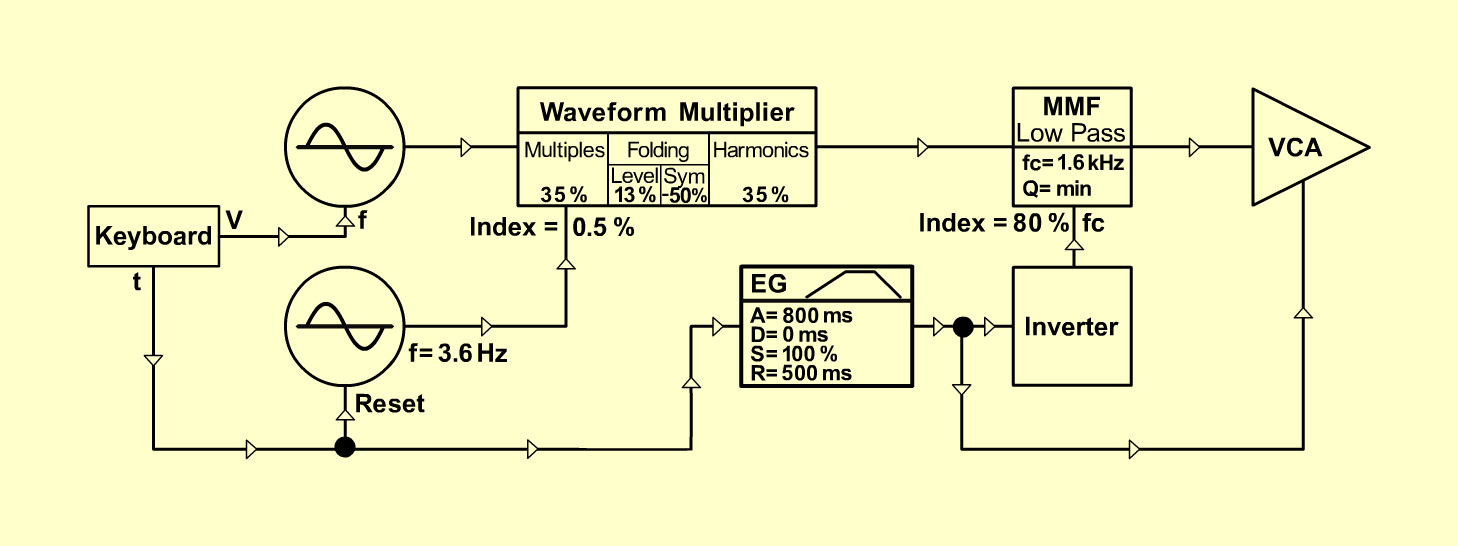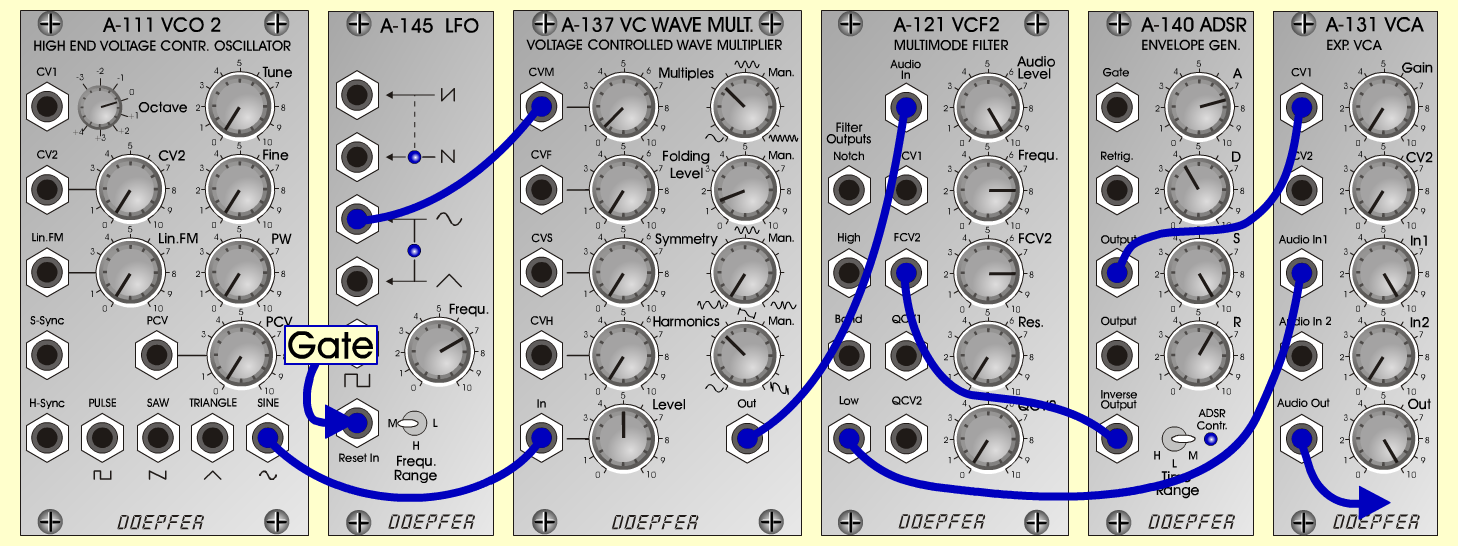Galactic-ViolSynth- P a dD o e p f e r A - 100 Connections: Settings: A-111 (Sine) <=> A-137 (In)   A-137 (Out) <=> A-121 (Audio In)   A-145 (Sine) <=> A-137 (CVM)   A-121 (Low) <=> A-131 (Audio In)   A-140 (Output) <=> A-131 (CV 1)   A-140 (Inverse Output) <=> A-121 (FCV 2)   Gate <=> A-145 (Reset In) A-137 (CV Multiples = 0.5, Man. Multiples = 3.5,   Man. Folding Level = 1.3, Man. Symmetry = 0,   Man. Harmonics = 3.5, Level = 5)   A-121 (Audio Level = 10, Freq = 8, FCV 2 = 8, Res = 0)   A-145 (Frq = 7, Range = M)   A-140 (A = 7.5, D = 4, S = 10, R = 6, Range = M)   A-131 (Gain = 0, Audio In 1 = 10, Audio Out = 10) Josef MuellerSound samples Galactic-Viol (55 Hz) Galactic-Viol (119 Hz) Galactic-Viol (220 Hz) Galactic-Viol (440 Hz) Galactic-Viol (880 Hz) Galactic-Viol - without filter release (440 Hz)